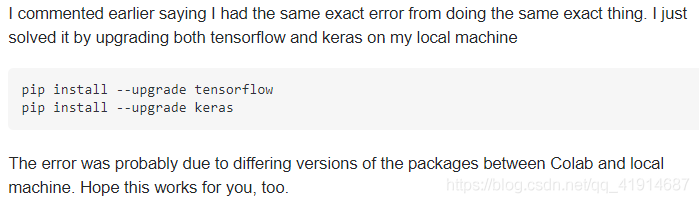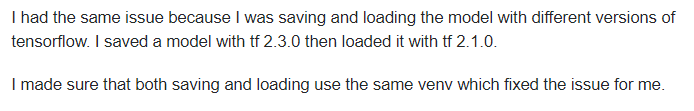# TypeError(‘Keyword argument not understood:‘, ‘***‘) in keras.models load_model

TypeError(‘Keyword argument not understood:’, ‘***’) in keras.models load_ model

1.Problem description

1. after training on Google colab, model.save (filepath)_ Save) and then use after saving
``````from keras.models import load_model
# Error: TypeError: ('Keyword argument not understood:', 'step_dim')``````

2.Solutions

1. method 1: confirm whether the versions of keras and TF are different twice. Someone’s solution: I only solved it by upgrading tensorflow and keras on the local computer at the same time``````pip install --upgrade tensorflow
``````

What he means is the version problem. After training on Google’s colab, the model is saved locally. When it is called locally, the loading model will report an error due to the different versions of the packages in the two environments
then you can adjust the version of the local related package.

Similar to the following answer, the version when the model is saved is inconsistent with the version when the model is loaded, which may cause this problem
then unify the versions``````import tensorflow as tf
import keras

print(keras.__version__)
print(tf.__version__)
``````

But mine is still read on the colab, and the environment is the same, so this method can’t solve my specific problem.

1. the general idea is that we start with models.load_ Model () reads the network and weight. Now, because of the keyword argument not understood in the custom model, we first build the model structure, and then model. Load_ Weights () reads weights, which can achieve our original purpose

1. at present, I use this method to solve the problem of re reading and importing the parameters of the network structure model of the user-defined model

I also have this problem I’ve tried a lot of methods and found that this method can be used

``````# first,build model
model = TextAttBiRNN(maxlen, max_features, embedding_dims).get_model()
model.compile(loss='binary_crossentropy',